Class and Object in Swift

View more Tutorials: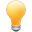Follow us on our fanpages to receive notifications every time there are new articles.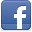Facebook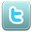Twitter

Swift is an inherited language from C and Objective-C, it is both a procedural language, both as a object oriented language. Class is a concept of the  object-oriented programming language. Class have properties and methods, naturally, method  is understood as a function of the class. From class you can create objects.
Swift using the class  keyword to declare a class.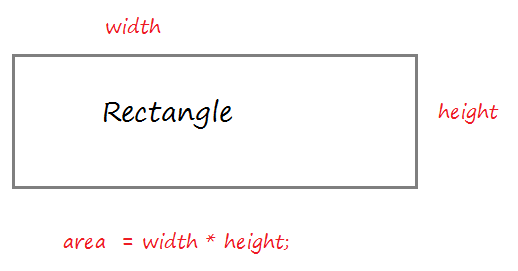Create a resource file Rectangle.swift:
Rectangle.swift

import Foundation

class Rectangle  {
// width property
var width: Int = 5;
// height property
var height: Int = 10;

// Default Constructor (No parameter)
// (Used to create instance).
init()  {

}
// Contructor with 2 parameters.
// (Used to create instance)
// self.width refers to the width property of the class
init (width: Int, height: Int)  {
self.width = width
self.height = height

}
// A method calculates the area of the rectangle.
func getArea()  -> Int  {
var area = self.width * self.height
return area
}
}
The code using Rectangle class to create the  object.
RectangleTest.swift

import Foundation

func test_rectangle()  {
print("Create a Rectangle rec1");
// Create Rectangle object
// via default constructor: init()
var rec1  = Rectangle()

// Print out width, height
print("rec1.width = \(rec1.width)")
print("rec1.height = \(rec1.height)")

// Call the method to calculate the area.
var area1 = rec1.getArea()
print("area1 = \(area1)")
print("---------")
print("Create a Rectangle rec2");

// Create Rectangle object
// via contructor with 2 parameters: init(Int,Int)
var rec2 = Rectangle(width: 10, height: 15)

// Print out width, height
print("rec2.width = \(rec2.width)")
print("rec2.height = \(rec2.height)")
// Call the method to calculate the area.
var area2 = rec2.getArea()
print("area2 = \(area2)")
}
Edit main.swift to test this example.
main.swift

import Foundation

test_rectangle()

Running the example:

Create a Rectangle rec1
rec1.width = 5
rec1.height = 10
area1 = 50
----------
Create a Rectangle rec2
rec2.width = 10
rec3.height = 15
area2 = 150
Now you should see the explanation of the Class, it's very important.
What happens when you create an object by default contructor?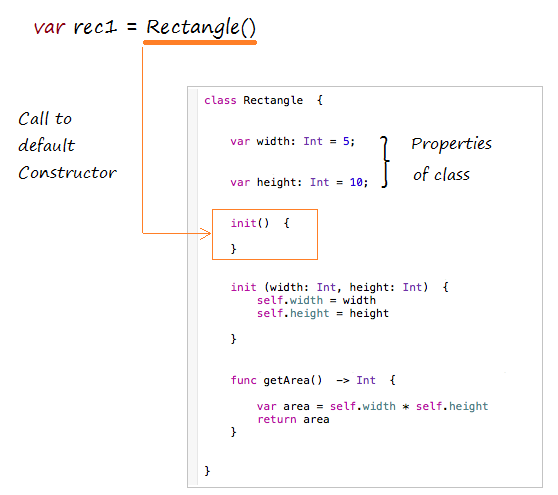Create a Rectangle object by contructor with 2 parameters.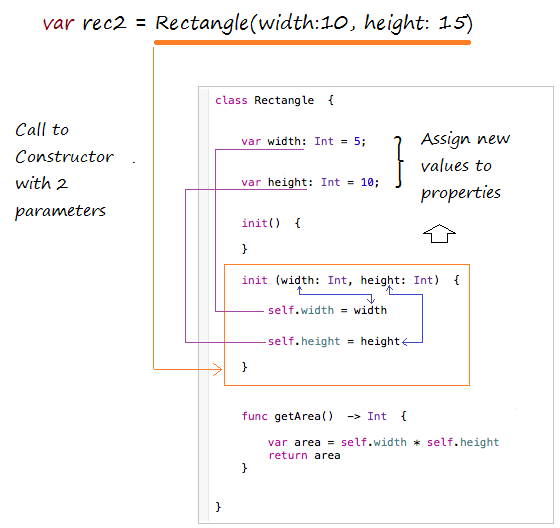2- Rule to call Constructor

Rules for Swift 2.1 to call function, method, constructor:
• First parameter pass to methods and functions should not have required argument labels.
• Other parameters pass to methods and functions should have required argument labels.
• All parameters pass to Constructors should have required argument labels.

3- Object Comparison operators

In Swift, when you create an object through Constructor will be a real entity is created in memory, it has a specified address.

An operator assigned AA object by an BB  object does not create new entity on the memory, it's just pointing to address of AA to BB's address.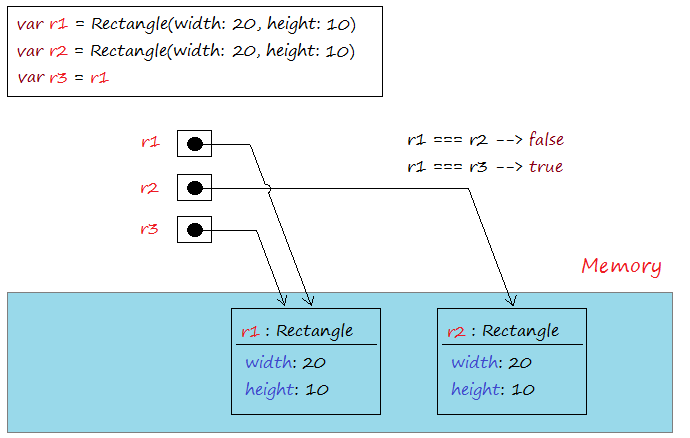=== Operator used to compare two objects that is pointing to, it returns true if the two objects refer to the same address in memory. Operators !== is used to compare the two addresses of two objects that is pointing to, it returns true if the two objects that point to two different addresses.
IdentifyOperator.swift

import Foundation

func identifyOperator()    {

// Create object: r1
var r1 = Rectangle(width: 20,height: 10)

// Create object: r2
var r2 = Rectangle(width: 20,height: 10)

var r3 = r1

var  b12:Bool =   r1 === r2
var  b13:Bool =   r1 === r3

print("r1 === r2 ?  \(b12)") // false
print("r1 === r3 ?  \(b13)") // true

var bb12: Bool = r1 !== r2
var bb13: Bool = r1 !== r3

print("r1 !== r2 ?  \(b12)") // true
print("r1 !== r3 ?  \(b13)") // false

}
Running the example:

r1 === r2 ? false
r1 === r3 ? true
r1 !== r2 ? false
r1 !== r3 ? true

View more Tutorials:

Maybe you are interested

These are online courses outside the o7planning website that we introduced, which may include free or discounted courses.

•iOS 11 / Swift 4 - Programming 101
•Make iPhone Apps Using Swift, Xcode and iOS8 - 7 Apps
•*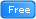* Swift for Beginners, Learn Apple's New Programming Language
•Learn MongoDB from scratch: Absolute beginner's introduction
•iOS 11 & Swift 4: From Beginner to Paid Professional™
•** Basic Swift 4 and iOS 11 - Free Preview
•Learning Web Application with Spring 5 and Angular 2
•C# Unity® and Swift 4 Masterclass: Make Games and Apps
•Swift - Advanced API's & Technique
•** Cloning Amazon, Netflix & Spotify
•Swift - Learn by doing
•iOS 11 Programming with SWIFT
•Basic Concepts of Web Development, HTTP and Java Servlets
•SQL Made Simple: SQL Server Queries for Non-Programmers
•iOS Development - Create 4 Quiz Apps with Swift 3 & iOS 10
•Swift Language Fundamentals
•Build a REST API with node.js, ExpressJS, and MongoDB
•Create an app like Twitter with Swift
•Beginner's Swift 4 & Unreal Engine - Learn Xcode, Make Games
•How to create a Flappy Bird inspired iPhone game in Swift 2
•iOS 11 & Swift 4.0 - A Beginner's Guide with Projects
•NodeJS in Action
•Master Java Web Services and RESTful API with Spring Boot
•iOS 10 Swift 3 - A Complete Practical Approach
•C#: The Complete Foundation!